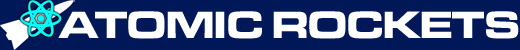## Instructions

Enter in density and any two of the remaining parameters. If one of the other inputs would produce an invalid dataset when combined with one already entered, it will be greyed out.

Calculator
Equivalent Density (required; see table)Kilograms/Cubic Meter
VolumeCubic Meters
MassKilograms
DiameterMeters
LengthMeters
Length to Diameter Ratio (decimal number greater than 2/3)Unitless
Table of Densities of Various Modules
Furnising LevelExampleEquivalent Density
Basic ModuleSundancer47.8 kg/m^3
Lightly FurnishedBA-33060 kg/m^3
Medium FurnishingHabitat Unit100 kg/m^3
Highly FurnishedLaboratory120 kg/m^3
Basic Rigid CanDestiny (Launch)118.5 kg/m^3

This calculator works via observations about the geometry of TransHab modules and the method of centroids for calculating volume.

The module consists of a rigid core and an inflated bladder, which seems to generally have triple the diameter of the rigid core. This volume can be broken down further into the endcone annular portions, which are approximately quarter-circular in cross-section, the endcone barrel sections, which are rectangular in cross section, and a central barrel section, also rectangular in cross section.

This volume can then be regrouped to produce a simpler arrangment with the same cross sectional areas and centroids. The centroid is the geometric center of ashape. For a shape like a rectangle or a circle, this is the middle point of the area. It's a bit more complicated for things like half-circles, but still can be found. Rotating this cross section around the center axis would thus yield the same volume as the TranHab module, and it turns out that the volume is equal to the area of the cross section times the distance the area's centroid travels (which is 2 times pi times the distance from the centroid to the axis of revolution for a full rotation). The geometry of module can be used to find the dimensions of each portion on this diagram (including accounting for skin thickness, which I calculate as approximately 0.3 meters) and the method of centroids used to calculate volume from that area as shown in the diagram below by summing the volume resulting from each section.

This core equation for volume is used to relate the diameter, ratio, and volume of the module. The length-to-diameter ratio is used to relate the diameter, ratio, and length of the module. The density is used to relate the mass and volume of the module. With these equations, density is always required to solve all parameters and generally two other inputs are needed, except for the following three pairings, which do are insufficient to solve for all parameters.

• Volume/Mass: over-constrains density, gives no second value for solving total volume equation to get a diameter or ratio.
• Length/Volume: gives no second value to solve to volume equation for diameter or ratio.
• Length/Mass: Density allows volume to be calculated, but then is identical to the case for Length/Volume.

### Caveats

This calculator assumes that the rigid core extends only the length of the inflatable portion. This is not quite true, since some Bigelow designs include a rigid docking portion extending out from the core, but since these end portions are not conastan in size relative to the module in question, I do not calculate their volume and they are not included in the final volume or mass total. If you wish to include them, this volume is probably best approximated as a normal "rigid can" module in terms of volume and mass.

### Warning

All calculated parameters are approximate. If you are using this calculator to engineer real modules: First, it's not built for that so check everything over a few times, and second, are you hiring interns?

## Atomic Rockets notices

This week's featured addition is SPIN POLARIZATION FOR FUSION PROPULSION

This week's featured addition is INsTAR

This week's featured addition is NTR ALTERNATIVES TO LIQUID HYDROGEN

### Support Atomic Rockets